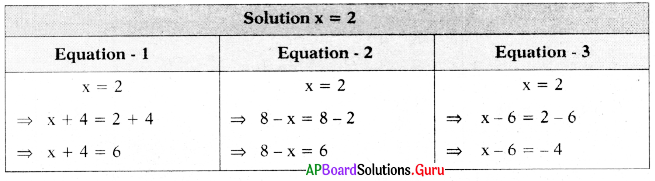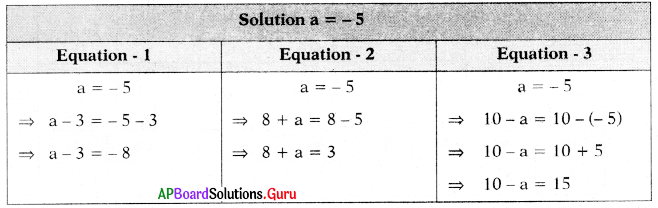SCERT AP 7th Class Maths Solutions Pdf Chapter 3 Simple Equations Ex 3.3 Textbook Exercise Questions and Answers.

## AP State Syllabus 7th Class Maths Solutions 3rd Lesson Simple Equations Exercise 3.3

Question 1.
Solve the following equations and check the result.
(i) 5x – 17 = 18
Given 5x – 17 = 18
⇒ 5x – 17 + 17 = 18 + 17 (Add 17 on both sides)
⇒ 5x = 35
⇒ $$\frac{5 x}{5}$$ = $$\frac{35}{5}$$ (Divide by 5 on both sides)
⇒ x = 7

Check:
Substitute x = 7 in 5x – 17 = 18
LHS = 5x – 17
= 5(7) – 17
= 35 – 17 = 18 = RHS
Hence verified.(ii) 29 – 7y = 1
Given 29 – 7y = 1
⇒ 29 – 7y – 29 = 1 – 29 (Subtract 29 on both sides)
⇒ – 7y = -28 .
⇒ $$\frac{-7 y}{-7}$$ = $$\frac{-28}{-7}$$ (Divide by – 7 on both sides)
⇒ y = 4

Check:
Substitute y = 4 in
29 – 7y = 1
LHS = 29 – 7y
= 29 – 7(4)
= 29 – 28 = 1 = RHS
Hence verified.

(iii) a – 2.3 = 1.5
Given a – 2.3 = 1.5
⇒ a – 2.3 + 2.3 = 1.5 + 2.3 (Add 2.3 ort both sides)
⇒ a = 3.8

Check:
Substitute a = 3.8 in a – 2.3 =1.5
LHS = a – 2.3
= 3.8 – 2.3
= 1.5 = RHS
Hence verified.

(iv) b + 3$$\frac{1}{2}$$ = $$\frac{7}{4}$$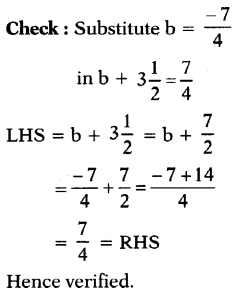(v) $$\frac{7 p}{10}$$ + 9 = 15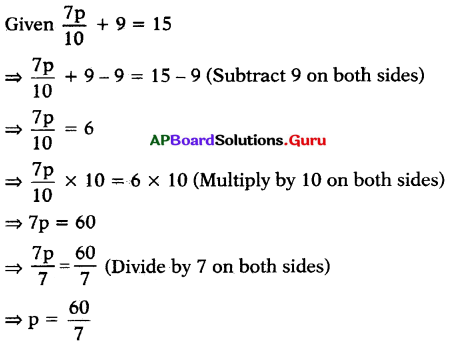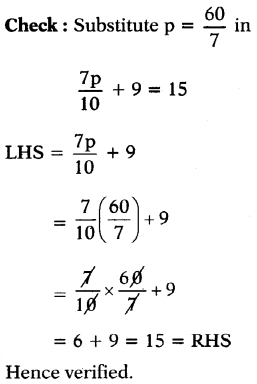(vi) 6(q – 5) = 42.
Given 6(q – 5) = 42
⇒ $$\frac{6(q-5)}{6}$$ = $$\frac{42}{6}$$ (Divide by 6 on both sides)
⇒ q – 5 = 7
⇒ q – 5 + 5 = 7 + 5 (Add 5 on both sides)
⇒ q = 12

Check:
Substitute q = 12 in
6(q – 5) = 42
LHS = 6(q – 5)
= 6(12 – 5)
= 6(7) = 42 = RHS
Hence verified.

(vii) – 3(m + 5) + 1 = 13
Given – 3(m + 5)4-1 = 13
⇒ – 3(m + 5)+ 1 – 1 = 13 – 1 (Subtract 1 on both sides)
⇒ – 3(m + 5) = 12
⇒ $$\frac{-3(m+5)}{-3}$$ = $$\frac{12}{-3}$$ (Divide by – 3 on both sides)
⇒ m + 5 = – 4
⇒ m + 5 – 5 = – 4 – 5 (Subtract 5 on both sides)
⇒ m = – 9

Check:
Substitute m = – 9 in – 3(m + 5) + 1 = 13
LHS = – 3(m + 5) + 1
= – 3(- 9 + 5) + 1 = (- 3 × – 4) + 1
= + 12 + 1 = 13 = RHS
Hence verified.(viii) $$\frac{n}{2}+\frac{n}{3}+\frac{n}{5}$$ = 31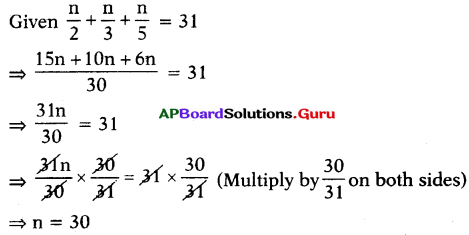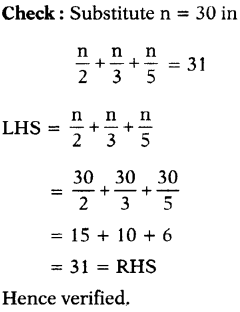Question 2.
Solve the following equations and check the result.

(i) 3(p – 7) – 4 = 5
Given 3(p – 7) – 4 = 5
⇒ 3(p – 7) – 4 + 4 = 5 + 4 (Add 4 on both sides)
⇒ 3(p – 7) = 9
⇒ $$\frac{3(p-7)}{3}$$ = $$\frac{9}{3}$$ (Divide by 3 on both sides)
⇒ p – 7 = 3
⇒ p – 7 + 7 = 3 + 7 (Add 7 on both sides)
⇒ p = 10

Check:
Substitute p = 10 in
3(p – 7) – 4 = 5
LHS = 3(p – 7) – 4.
= 3(10 – 7) – 4.
= 3 × 3 – 4
= 9 – 4 = 5 = RHS
Hence verified.

(ii) 5(q – 3) – 3(q – 2) = 0
Given 5(q – 3) – 3(q – 2) = 0
⇒ 5q – 15 – 3q + 6 = 0 (Distributive property)
⇒ 2q – 9 = 0 .
⇒ 2q – 9 + 9 = 0 + 9 (Add 9 on both sides)
⇒ 2q = 9
⇒ $$\frac{2 \mathrm{q}}{2}$$ = $$\frac{9}{2}$$ (Divide by 2 on both sides)
⇒ q = $$\frac{9}{2}$$

Check : Substitute q = $$\frac{9}{2}$$ in
5(q – 3) – 3(q – 2) = 0
LHS = 5(q – 3) – 3(q – 2)
= 5$$\left(\frac{9}{2}-3\right)$$ – 3$$\left(\frac{9}{2}-2\right)$$
= 5$$\left(\frac{9-6}{2}\right)$$ – 3$$\left(\frac{9-4}{2}\right)$$
= 5 × $$\frac{3}{2}$$ – 3 × $$\frac{5}{2}$$
= $$\frac{15}{2}-\frac{15}{2}$$ = 0 = RHS
Hence verified.(iii) 4x – 0.3x – 1.2 = 0.6
Given 0.4x – 0.3x – 1.2 = 0.6
⇒ 0.1 x – 1.2 = 0.6
⇒ 0.1 x – 1.2 + 1.2 = 0.6 + 1.2 (Add 1.2 on both sides)
⇒ 0.1 x = 1.8
⇒ $$\frac{1 \mathrm{x}}{10}$$ = $$\frac{18}{10}$$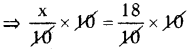(Multiply by 10 on both sides)
⇒ x = 18

Check:
Substitute x = 18 in
0.4x – 0.3x – 1.2 = 0.6
LHS = 0.4x – 0.3x – 1.2
= 0.4(18) – 0.3(18) – 1.2
= 7.2 – 5.4 – 1.2
= 7.2 – 6.6 = 0.6 = RHS
Hence verified.

(iv) 4(3y + 4) = 7.6
Given 4(3y + 4) = 7.6.
⇒ $$\frac{4(3 y+4)}{4}$$ = $$\frac{7.6}{4}$$ (Divide by 4 on both sides)
⇒ 3y + 4 = 1.9
⇒3y + 4 – 4= 1.9 – 4 (Subtract 4 on both sides)
⇒ 3y = – 2.1
⇒ $$\frac{3 y}{3}$$ = – $$\frac{2.1}{3}$$ (Divide by 3 on both sides)
⇒ y = – 0.7

Check:
Substitute y = – 0.7 in
4(3y + 4) = 7.6
LHS = 4(3y + 4)
= 4[3 (- 0.7) + 4]
= 4[- 2.1 + 4]
= 4 × 1.9 = 7.6 = RHS
Hence verified.

(v) 20 – (2r – 5) = 25
Given 20 – (2r – 5) = 25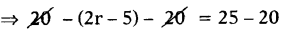(Subtract 20 on both sides)
⇒ – (2r – 5) = 5
⇒ – (2r -5) × – 1 = 5 × – 1
(Multiply by – 1 on both sides)
⇒ 2r – 5 = 5
⇒ 2r – 5 + 5= – 5 + 5 (Add 5 on both sides)
⇒ 2r = 0
⇒ $$\frac{2 \mathrm{r}}{2}$$ = $$\frac{0}{2}$$ (Divide by 2 on both sides)
⇒ r = 0

Check:
Substitute r = 0 in
20 – (2r – 5) = 25
LHS = 20 – (2r – 5)
= 20 – [2(0) – 5]
= 20 – [0 – 5]
= 20 – (- 5)
= 20 + 5 = 25 = RHS
Hence verified.(vi) 3(5 – t) – 2(t – 2) = – 1
Given 3(5 – t) – 2(t – 2) = – 1
⇒ 15 – 3t – 2t + 4 .= – 1 (Distributive property)
⇒ 19 – 5t = – 1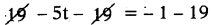(Subtract 19 on both sides)
⇒ – 5t = – 20
⇒ $$\frac{-5 t}{-5}$$ = $$\frac{-20}{-5}$$ (Divide by – 5 on both sides)
⇒ t = 4

Check:
Substitute t = 4 in
3(5 -1) – 2(t – 2) = – 1
LHS = 3(5 – t) – 2(t – 2)
= 3(5 – 4) – 2(4 – 2)
= 3(1) – 2(2)
= 3 – 4 = – 1 = RHS
Hence verified.

(vii) 3(2k + 1) – 2(k – 5) – 5(5 – 2k) = 16
Given 3(2k + 1) – 2(k T 5) – 5(5 – 2k) = 16
⇒ 6k + 3-2k + 10-25 + 10k = 16 (Distributive property)
⇒ 14k – 12 = 16
⇒ 14k – 12 + 12 – 16 + 12 (Add 12 on both sides)
⇒ 14k = 28
⇒ $$\frac{14 \mathrm{k}}{14}$$ = $$\frac{28}{14}$$ (Divide by 14 on both sides)
⇒ k = 2

Check:
Substitute k = 2 in
3(2k + 1) – 2(k – 5) – 5(5 – 2k) = 16
LHS = 3(2k + 1) – 2(k – 5) – 5(5 – 2k)
= 3[2 × 2 + 1] – 2[2 – 5] – 5[5 – 2 × 2]
= 3[4 + 1] – 2(- 3) – 5(5 – 4)
= 15 + 6 – 5 – 16 = RHS
Hence verified.(viii) $$\frac{3 \mathrm{~m}}{4}$$ – 5m – $$\frac{3}{4}$$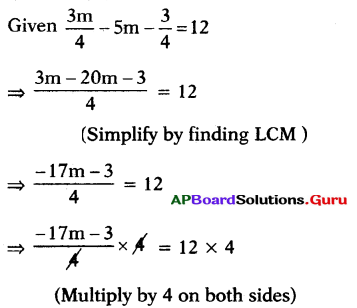⇒ – 17m – 3 = 48
⇒ – 17m – 3 + 3 =48 + 3 (Add 3 on both sides)
⇒ – 17m = 51
⇒ $$\frac{-17 \mathrm{~m}}{-17}$$ = $$\frac{51}{-17}$$ (Divide by – 17 on both sides)
⇒ m = – 3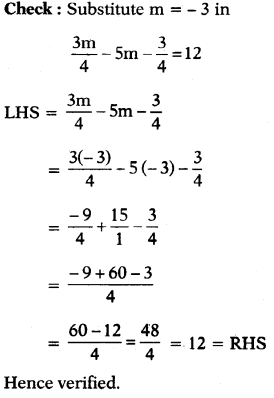(ix) $$\frac{4 n}{5}+\frac{n}{4}-\frac{n}{2}=\frac{11}{10}$$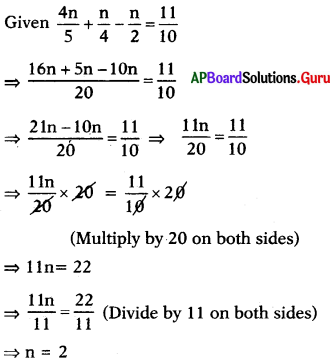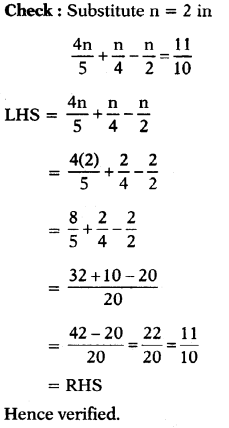(x) $$\frac{x}{2}-\frac{4}{5}+\frac{x}{5}+\frac{3 x}{10}=\frac{1}{5}$$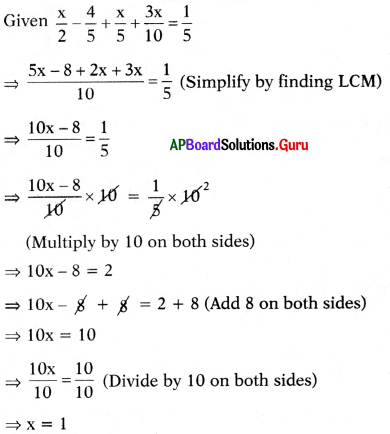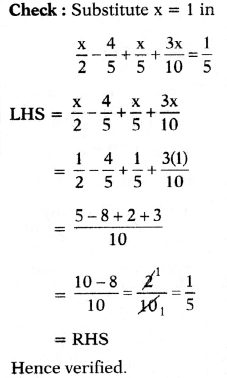Question 3.
Write any three equivalent equations having the solution x = 2.